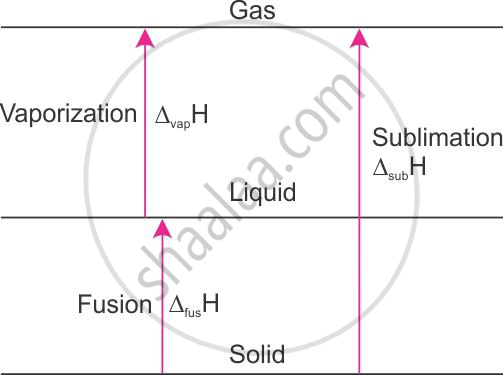# How is Enthalpy of Sublimation Related to Enthalpy of Fusion and Enthalpy of Vaporization - Chemistry

How is enthalpy of sublimation related to enthalpy of fusion and enthalpy of vaporization?

#### Solution

Relation of enthalpy of sublimation with enthalpy of fusion and enthalpy of vaporisation:The enthalpy of sublimation of ice at 00C and 1 atm pressure is 51.08 kJ mol−1.H2O(s) → H2O(g), ΔH = 51.08 kJ mol−1 at 00C.

When solid is converted to vapour, either in one step or two steps, the solid first gets converted to the liquid state and then to the vapour state. The enthalpy change remains the same. This is because enthalpy is a state function.

For example,
H2O(s) → H2O(l), Δfus H = +6.01 kJ mol−1 at 0°C
H2O(l) → H2O(g), Δvap H = +45.07 kJ mol−1 at 0°C

_____________________________________

H2O(s) → H2O(g), Δ H = 51.08 kJ mol−1 at 0°C

Therefore, it follows that
Δsub H = Δfus H + Δvap H

Concept: Chemical Thermodynamics and Energetic - Internal Energy and Enthalpy
Is there an error in this question or solution?